Web www.handwritingforkids.com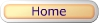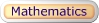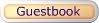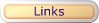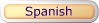### Basic Math 2 - Subtraction (Horizontal)

This printable basic math subtraction worksheet generator will provides plenty of math practice, boost test scores and prepare the child/children to meet the Kindergarten, Grade 1, and Grade 2 curriculum standards. This math worksheet maker will create a horizontal math subtraction, 1 digit by 1 digit (1x1) and 2 digits by 1 digit (2x1) equations.

minuend
subtrahend
difference12 - 4 = 8

 See sample math worksheets below:

1. Select the number of math problems per page. (Default: 1 - 18)
2. Select Type of Math Problems: (Default: Difference 0 to 10)
3. Click on submit to make your personalized horizontal math subtraction worksheet.
4. Option: Page back and click Submit to create a different set of math problems.

Basic Math 2 - Subtraction Worksheet Maker (Horizontal)

 Enter the Number of Math Problems per page: Select a range 1 - 3 1 - 6 1 - 9 1 - 12 1 - 15 1 - 18 Default: 1 - 18 Type of Math Problems: Select a Type of Math Difference 0 to 5 Difference 5 to 10 Difference 0 to 10 Difference 0 to 18 Difference 10 to 18 Default: Difference 0 to 10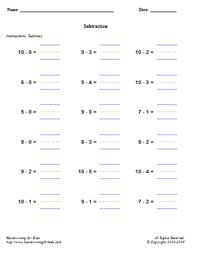Basic Handwriting for Kids Free for Non-Profit Use Ms. Readman, Rockford, IL Revised: